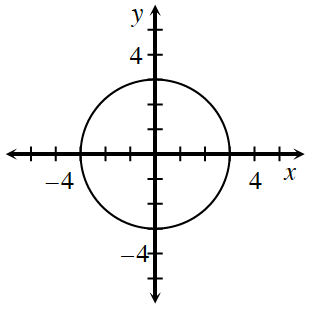### Home > INT2 > Chapter 10 > Lesson 10.1.1 > Problem10-7

10-7.Examine the graph of the circle at right.

1. What is the equation of the circle?

Sketch a right triangle from the origin as in $10-1$ and label the hypotenuse with the value for the radius.

The equation of a circle is directly derived from the Pythagorean Theorem.

2. On graph paper, sketch the graph of  $x^2 + y^2 = 81$. What is the radius?

The circle with equation $x^2+y^2=36$ has a radius of $6$.